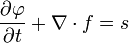# The most beautiful formulae in maths & science

Pythagorean
Gold Member
It can be represented by either $\nabla^2$ or $\Delta$ and technically it should be $\Delta f$ but I left it out because I am in fact quite mad/evil.

yay, new notation. I was as equally excited by the d'Alembertian, square as it is.

my simpleton contribution to this thread:

I was very excited when (during the course of my education)

$$F = ma$$

evolved into
$$F = m \frac{d^2x}{dt^2}$$

and further to
$$F = \frac{dp}{dt}$$

I really like the continuity equation too:Last edited:
EL
I was very excited when (during the course of my education)

$$F = ma$$

evolved into
$$F = m \frac{dx}{dt}$$

Wow, so F=ma has evolved into F=mv...Pythagorean
Gold Member

Stokes's theorem: $$\int_Md\omega = \int_{\partial M}\omega$$
Elegant, concise, obvious once the components are properly defined, and pops up in cute places in classical physics and differential geometry.

One of the most ridiculous and ugliest equation ever:

$$N = R^* \times f_p \times n_e \times f_l \times f_i \times f_c \times L$$

This is the 'Drake equation' where N is the number of civilizations in our galaxy with which we might hope to be able to communicate.

and some estimates even give the value as '10'...

Last edited:
EL
One of the most ridiculous and ugliest equation ever:

$$N = R^* \times f_p \times n_e \times f_l \times f_i \times f_c \times L$$

This is the 'Drake equation' where N is the number of civilizations in our galaxy with which we might hope to be able to communicate.

and some estimates even give the value as '10'...
True! There's no reason to have a special name for that kind of equations.

True! There's no reason to have a special name for that kind of equations.

Equations should be equation, singular. I'm only pointing this out because you asked.

One of the most ridiculous and ugliest equation ever:

$$N = R^* \times f_p \times n_e \times f_l \times f_i \times f_c \times L$$

This is the 'Drake equation' where N is the number of civilizations in our galaxy with which we might hope to be able to communicate.

and some estimates even give the value as '10'...

Well as I said on the thread where I used this it's meant to be a starting point for discussion, where people can suggest their own values; it's entirely hypothetical; it's parameters are meant to be unknowable, and any conclusions based on it are by these criteria, purely speculative. So I would say yes its ugly but I don't think it's ridiculous, after all it is meant purely to encourage discussion on life in the Universe, not as an accurate measure of such.